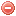# Description

The Fosberg Fire Weather Index ($$FFWI$$) is a fire danger index developed by Fosberg (1978). It is based upon equilibrium moisture content and wind speed, and requires hourly observations of temperature, relative air humidity and wind speed as input data (Fosberg, 1978, Goodrick 2002, Sharples 2009a).

It was designed for assessing the impacts of small-scale/short term weather variations on fire potential and is highly sensitive to changes in fine fuel moisture (Goodrick 2002, Crimmins 2005).

The $$FFWI$$ has been found to be correlated with fire occurrence in the northeastern and southwestern United States (Haines et al. 1983, Roads et al. 1997, Sharples 2009a).

# Formula

The $$FFWI$$ formulation is divided into a fuel moisture component, corresponding to the equilibrium moisture content defined by Simard (1968), and a rate of spread component based on the Rothermel (1972) model (Goodrick 2002):

$FFWI=\dfrac{\eta\cdot{\sqrt{1+U^2}}}{0.3002}$

where $$U$$ is the wind speed [mph] and $$\eta$$ the moisture damping coefficient which is calculated as follows:

$\eta=1-2\cdot\bigg(\dfrac{EMC}{30}\bigg)+1.5\cdot\bigg(\dfrac{EMC}{30}\bigg)^{2}-0.5\cdot\bigg(\dfrac{EMC}{30}\bigg)^3$

where $$EMC$$ is the equilibrium moisture content [mm].

NB1: the $$FFWI$$ was originally supposed to be calculated on an hourly basis.

NB2: fuel bed properties (surface area to volume ratio and moisture of extinction) are assumed to be constant over space and time, with a surface area to volume ratio of 3000 feet-1, and moisture of extinction of 30 percent.

No particular values or conditions are required when starting the $$FFWI$$ calculation.

# Modifications

The fact that the $$FFWI$$ does not take rainfall into account was considered as problematic, in particular for capturing spatial variations in fire potential in regions where spatial variability of rainfall is important (Goodrick 2002). Therefore, a rainfall component, in the form of a "fuel availability" factor ($$FAF$$) was added by Goodrick (2002) to the $$FFWI$$ in order to take the impact of drought on fuels into account.

This fuel availability factor is a function of the $$KBDI$$ (NB: in hundredths of inches) and is calculated as follows:

$FAF=0.000002\cdot{KBDI^2}+0.72$

The modified $$FFWI$$ ($$mFFWI$$) is then obtained by multiplying the fuel availability factors with the $$FFWI$$:

$\mathit{mFFWI}=\mathit{FAF}\cdot{\mathit{FFWI}}$

The $$mFFWI$$ is supposed to be calculated on a hourly basis. Thus the meteorological data used for its calculation are the meteorological data at the time of the basic weather observation.

No particular values or conditions are required when starting the index calculation. However, as the $$mFFWI$$ includes the $$KBDI$$ in its formulation, the starting conditions of the $$KBDI$$ have to be met, i.e. the soil layer has to be saturated with water, e.g. after a period of abundant rainfall, e.g. 6 or 8 [in] in a period of a week. The $$KBDI$$ component takes the starting value of 0.

Original publication:
Fosberg (1978)

Other publications:
Simard (1968)
Rothermel (1972)
Haines et al. (1983)
Goodrick (2002)
Crimmins (2005)
Sharples et al. (2009a)

### Bibliography SearchNo results!

No results were found. Are you sure you searched for a tag?

### Symbols

 Variable Description Unit $$T$$ air temperature °C $$T_{dew}$$ dew point temperature °C $$H$$ air humidity % $$P$$ rainfall mm $$U$$ windspeed m/s $$w$$ days since last rain (or rain above threshold) d $$rr$$ days with consecutive rain d $$\Delta t$$ time increment d $$\Delta{e}$$ vapor pressure deficit kPa $$e_s$$ saturation vapor pressure kPa $$e_a$$ actual vapor pressure kPa $$p_{atm}$$ atmospheric pressure kPa $$PET$$ potential evapotranspiration mm/d $$r$$ soil water reserve mm $$r_s$$ surface water reserve mm $$EMC$$ equilibrium moisture content % $$DF$$ drought factor - $$N$$ daylight hours hr $$D$$ weighted 24-hr average moisture condition hr $$\omega$$ sunset hour angle rad $$\delta$$ solar declination rad $$\varphi$$ latitude rad $$Cc$$ cloud cover Okta $$J$$ day of the year (1..365/366) - $$I$$ heat index - $$R_n$$ net radiation MJ⋅m-2⋅d-1 $$R_a$$ daily extraterrestrial radiation MJ⋅m-2⋅d-1 $$R_s$$ solar radiation MJ⋅m-2⋅d-1 $$R_{so}$$ clear-sky solar radiation MJ⋅m-2⋅d-1 $$R_{ns}$$ net shortwave radiation MJ⋅m-2⋅d-1 $$R_{nl}$$ net longwave radiation MJ⋅m-2⋅d-1 $$\lambda$$ latent heat of vaporization MJ/kg $$z$$ elevation m a.s.l. $$d_r$$ inverse relative distance Earth-Sun - $$\alpha$$ albedo or canopy reflection coefficient - $$\Delta$$ slope of the saturation vapor pressure curve kPa/°C $$Cc$$ cloud cover eights $$ROS$$ rate of spread m/h $$RSF$$ rate of spread factor - $$WF$$ wind factor - $$WRF$$ water reserve factor - $$FH$$ false relative humidity - $$FAF$$ fuel availability factor - $$PC$$ phenological coefficient -

 Suffix Description $$-$$ mean / daily value $$_{max}$$ maximum value $$_{min}$$ minimum value $$_{12}$$ value at 12:00 $$_{13}$$ value at 13:00 $$_{15}$$ value at 15:00 $$_{m}$$ montly value $$_{y}$$ yearly value $$_{f/a}$$ value at fuel-atmosphere interface $$_{dur}$$ duration $$_{soil}$$ value at soil level

 Constant Description $$e$$ Euler's number $$\gamma$$ psychrometric constant $$G_{SC}$$ solar constant $$\sigma$$ Stefan-Bolzmann constant